Next: C.4 Contraction of input Up: C. Proofs Previous: C.2 The eigenvalue equation

# C.3 Estimate of error accumulation

This section shows that the expectation value of the square error of the anticipated sensory input increases only linearly with the number of anticipation steps (Hoffmann and Möller, 2004). Let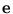be the error of the feed-forward output after a single step.is a vector with one component for each output component. We assume that the probability distribution of this error is independent of the input to the network. Thus, all errors are independent of each other. In addition, we assume that the error for each output component has zero mean and the same standard deviation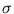.

On this basis, we compute the expectation value of the square error. The total error of the chain output is the sum of the errors of the outputs of each link. To illustrate this, think of each correct transformation at one link as a line in a d-dimensional space, with d equal to the number of output components (figure C.1).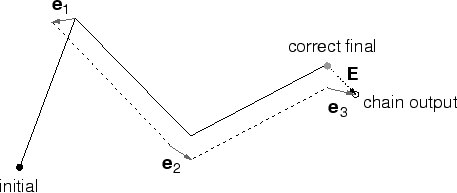A line connects an input point with an output point (of the transformation). The error at link i can be drawn as an arrow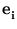at the end of a line (output point). This will result in a different starting point for the next line. If the error is smallC.1 and the transformation function sufficiently smooth, we can approximate that the displacement of the starting point does not change the direction and length of the next line, which is the correct transformation at the new starting point. Thus, the displacement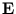of the final point is the sum of the vectorial errors of each stage. Therefore, given l links, the total error E can be written as

 E =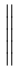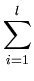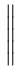. (C.10)

We compute the expectation value of E2,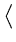E2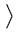=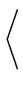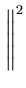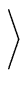. (C.11)

Doing the square operation on the sum givesE2=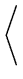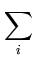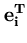+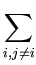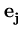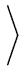, (C.12)

and using the linear property of the expectation value results inE2=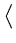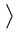+=. (C.13)

The last term vanishes becauseand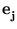are independent random variables, for i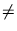j, and each variable has zero mean. The remainder is a sum over the variances for each link and dimension. Therefore,E2= l d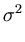. (C.14)

Thus, the expectation value of the square error increases only linearly with the chain length.Next: C.4 Contraction of input Up: C. Proofs Previous: C.2 The eigenvalue equation
Heiko Hoffmann
2005-03-22